# 1、数据介绍

``````import pandas as pd
import matplotlib.pyplot as plt

#ChinaBank.index = pd.to_datetime(ChinaBank.index)
sub = ChinaBank['2014-01':'2014-06']['Close']
train = sub.ix['2014-01':'2014-03']
test = sub.ix['2014-04':'2014-06']
plt.figure(figsize=(10,10))
print(train)
plt.plot(train)
plt.show()
``` 可思数据sykv.com,sykv.cn ```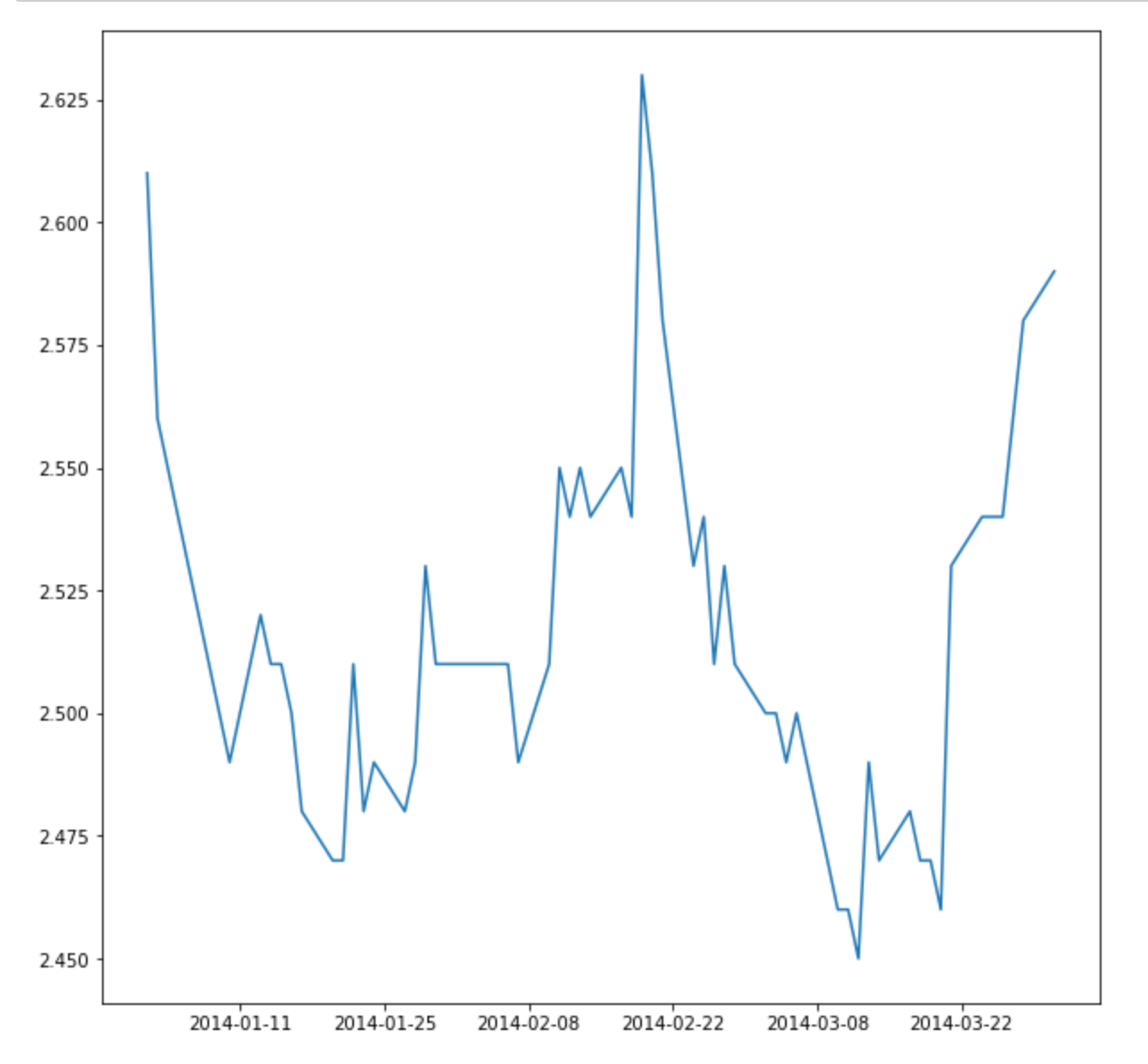# 2、时间序列平稳性

## 2.2 差分法

``````ChinaBank['Close_diff_1'] = ChinaBank['Close'].diff(1)
ChinaBank['Close_diff_2'] = ChinaBank['Close_diff_1'].diff(1)
fig = plt.figure(figsize=(20,6))
ax1.plot(ChinaBank['Close'])
ax2.plot(ChinaBank['Close_diff_1'])
ax3.plot(ChinaBank['Close_diff_2'])
plt.show()
``` 可思数据-数据挖掘,智慧医疗,机器视觉,机器人sykv.com ```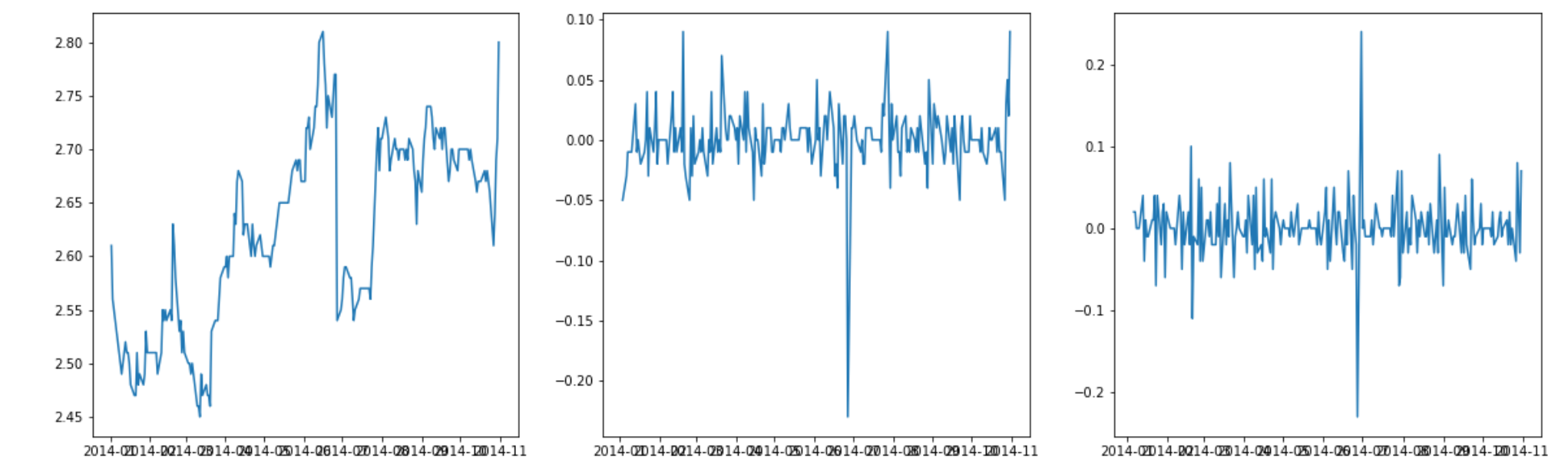# 3、ARIMA模型介绍

## 3.1 自回归模型AR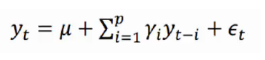1、自回归模型是用自身的数据进行预测
2、时间序列数据必须具有平稳性
3、自回归只适用于预测与自身前期相关的现象 可思数据sykv.com,sykv.cn

## 3.2 移动平均模型MA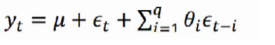## 3.3 自回归移动平均模型ARMA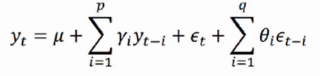# 4、建立ARIMA模型的过程

## 4.1 模型识别和定阶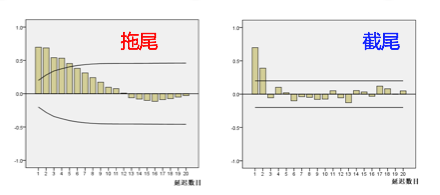1）在最初的d阶明显大于2倍标准差范围
2）之后几乎95%的(偏)自相关系数都落在2倍标准差范围以内 可思数据sykv.com,sykv.cn
3）且由非零自相关系数衰减为在零附近小值波动的过程非常突然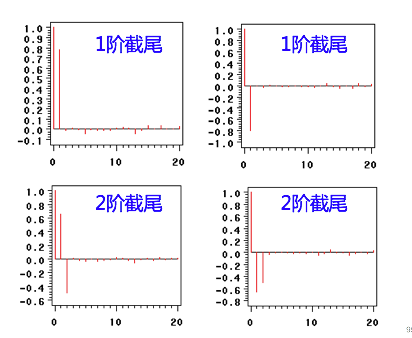1）如果有超过5%的样本(偏)自相关系数都落入2倍标准差范围之外 内容来自可思数据sykv.com
2）或者是由显著非0的(偏)自相关系数衰减为小值波动的过程比较缓慢或非常连续。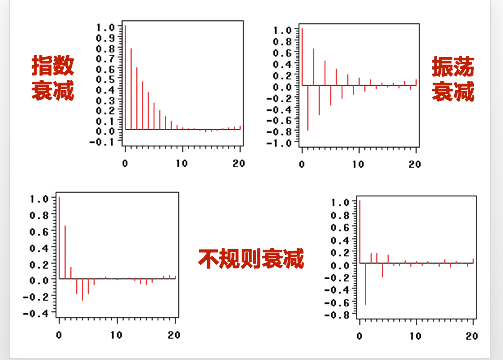p，q阶数的确定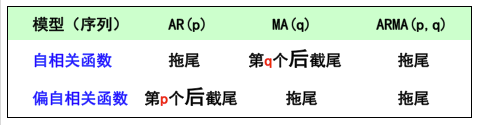``````import statsmodels.api as sm
fig = plt.figure(figsize=(12,8))

fig = sm.graphics.tsa.plot_acf(train, lags=20,ax=ax1)
ax1.xaxis.set_ticks_position('bottom')
fig.tight_layout()

fig = sm.graphics.tsa.plot_pacf(train, lags=20, ax=ax2)
ax2.xaxis.set_ticks_position('bottom')
fig.tight_layout()
plt.show()
``` 可思数据sykv.com ```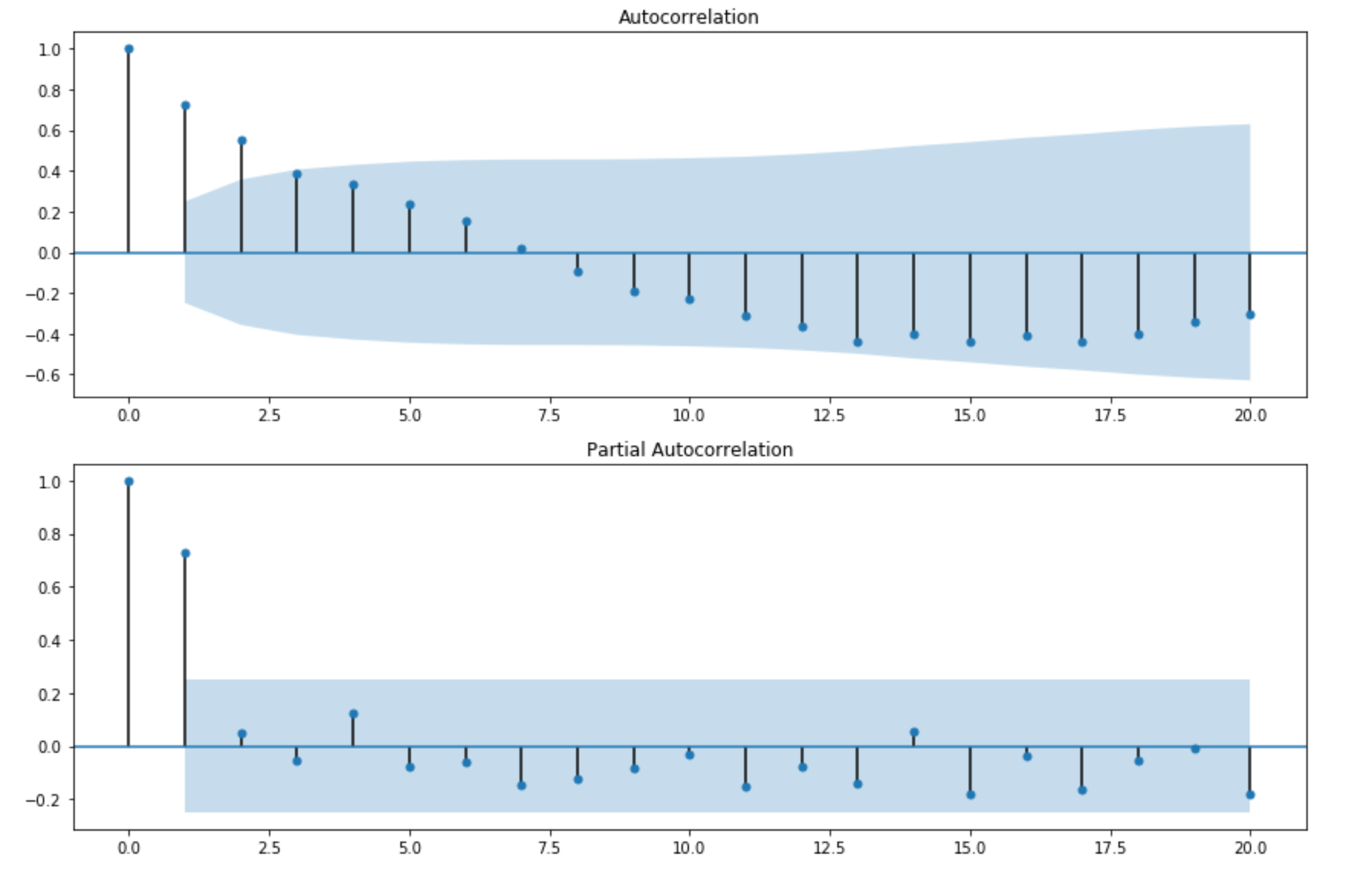## 4.2 参数估计

AIC准则
AIC准则全称为全称是最小化信息量准则（Akaike Information Criterion），计算公式如下：
AIC = =2 *（模型参数的个数）-2ln（模型的极大似然函数）

BIC准则
AIC准则存在一定的不足之处。当样本容量很大时，在AIC准则中拟合误差提供的信息就要受到样本容量的放大，而参数个数的惩罚因子却和样本容量没关系（一直是2），因此当样本容量很大时，使用AIC准则选择的模型不收敛与真实模型，它通常比真实模型所含的未知参数个数要多。BIC（Bayesian InformationCriterion）贝叶斯信息准则弥补了AIC的不足，计算公式如下：

BIC = ln(n) * (模型中参数的个数) – 2ln(模型的极大似然函数值)，n是样本容量 可思数据sykv.com,sykv.cn

``````#遍历，寻找适宜的参数
import itertools
import numpy as np
import seaborn as sns

p_min = 0
d_min = 0
q_min = 0
p_max = 5
d_max = 0
q_max = 5

# Initialize a DataFrame to store the results,，以BIC准则
results_bic = pd.DataFrame(index=['AR{}'.format(i) for i in range(p_min,p_max+1)],
columns=['MA{}'.format(i) for i in range(q_min,q_max+1)])

for p,d,q in itertools.product(range(p_min,p_max+1),
range(d_min,d_max+1),
range(q_min,q_max+1)):
if p==0 and d==0 and q==0:
results_bic.loc['AR{}'.format(p), 'MA{}'.format(q)] = np.nan
continue

try:
model = sm.tsa.ARIMA(train, order=(p, d, q),
#enforce_stationarity=False,
#enforce_invertibility=False,
)
results = model.fit()
results_bic.loc['AR{}'.format(p), 'MA{}'.format(q)] = results.bic
except:
continue
results_bic = results_bic[results_bic.columns].astype(float)

fig, ax = plt.subplots(figsize=(10, 8))
ax = sns.heatmap(results_bic,
ax=ax,
annot=True,
fmt='.2f',
)
ax.set_title('BIC')
plt.show()
``` 可思数据sykv.com,sykv.cn ```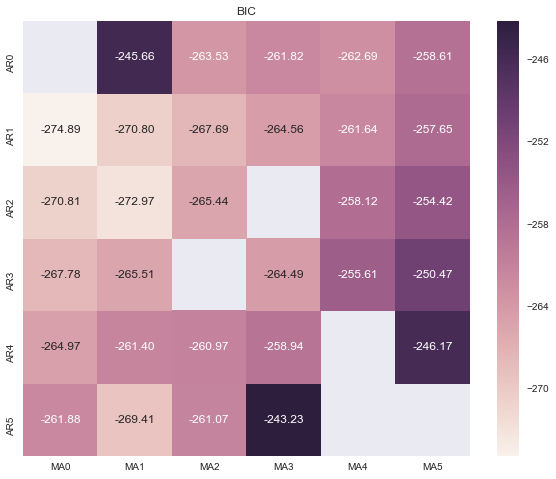``````train_results = sm.tsa.arma_order_select_ic(train, ic=['aic', 'bic'], trend='nc', max_ar=8, max_ma=8)

print('AIC', train_results.aic_min_order)
print('BIC', train_results.bic_min_order)
``` 内容来自可思数据sykv.com ```

``````AIC (1, 0)
BIC (1, 0)
``` 可思数据-数据挖掘,智慧医疗,机器视觉,机器人sykv.com ```

## 4.3 模型检验

1）检验参数估计的显著性（t检验）
2）检验残差序列的随机性，即残差之间是独立的

``````model = sm.tsa.ARIMA(train, order=(1, 0, 0))
results = model.fit()
resid = results.resid #赋值
fig = plt.figure(figsize=(12,8))
fig = sm.graphics.tsa.plot_acf(resid.values.squeeze(), lags=40)
plt.show()
``` 可思数据-AI,sykv.com智能驾驶,人脸识别,区块链,大数据 ```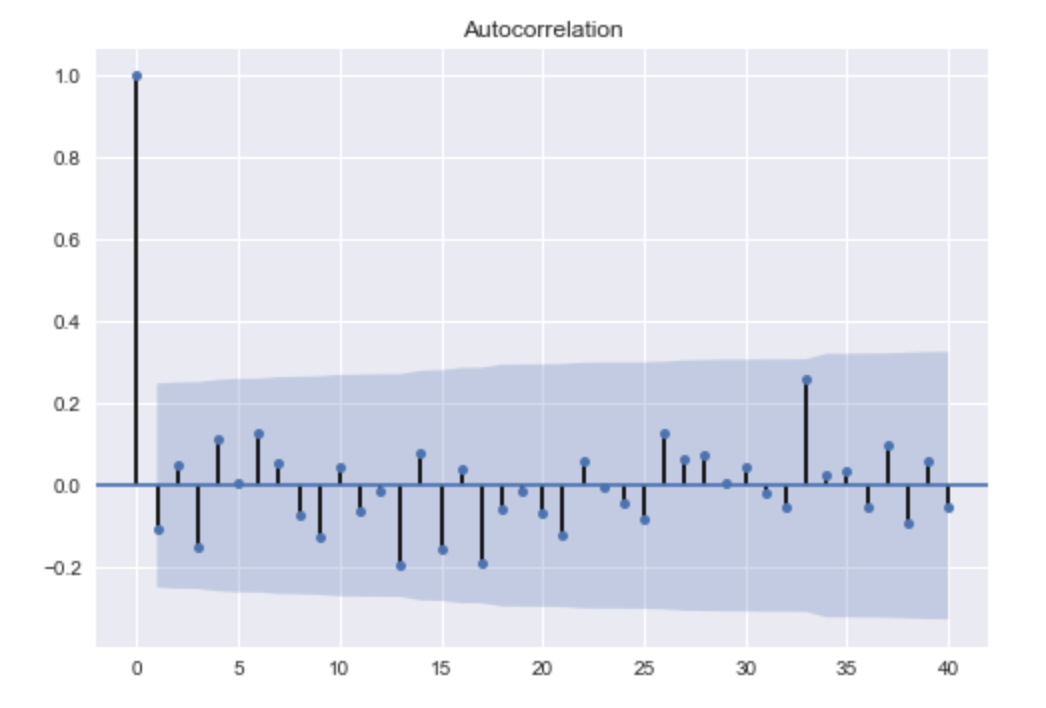## 4.4 模型预测

``````model = sm.tsa.ARIMA(sub, order=(1, 0, 0))
results = model.fit()
predict_sunspots = results.predict(start=str('2014-04'),end=str('2014-05'),dynamic=False)
print(predict_sunspots)
fig, ax = plt.subplots(figsize=(12, 8))
ax = sub.plot(ax=ax)
predict_sunspots.plot(ax=ax)
plt.show()
``` 内容来自可思数据sykv.com ```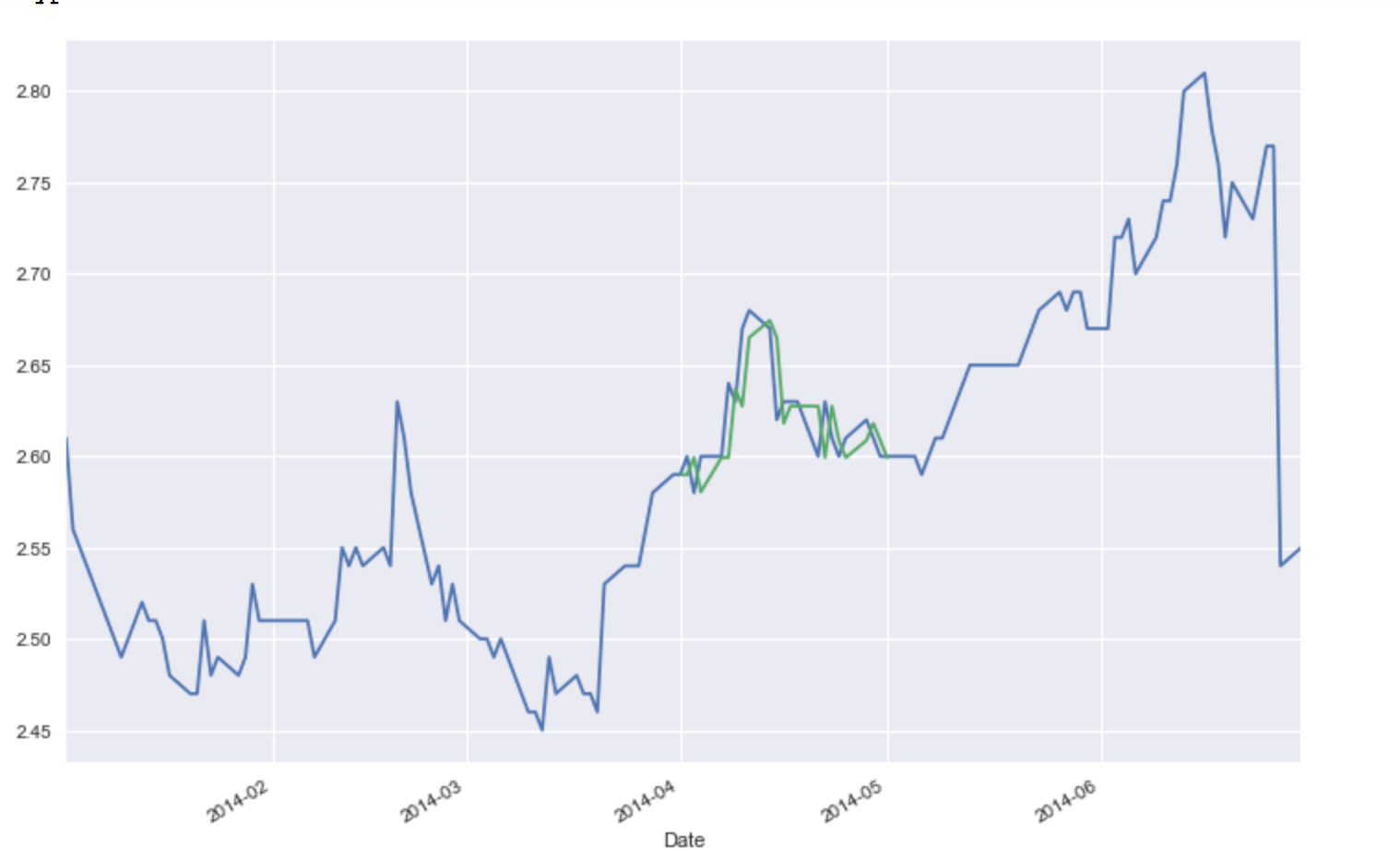``````results.forecast()
``` 内容来自可思数据sykv.com ```

# 参考文献

https://blog.csdn.net/weixin_41988628/article/details/83149849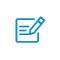扫码入群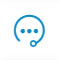咨询反馈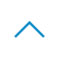返回顶部